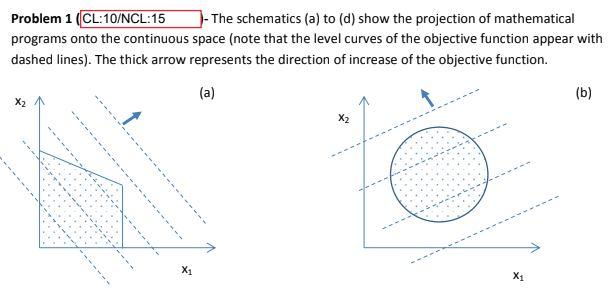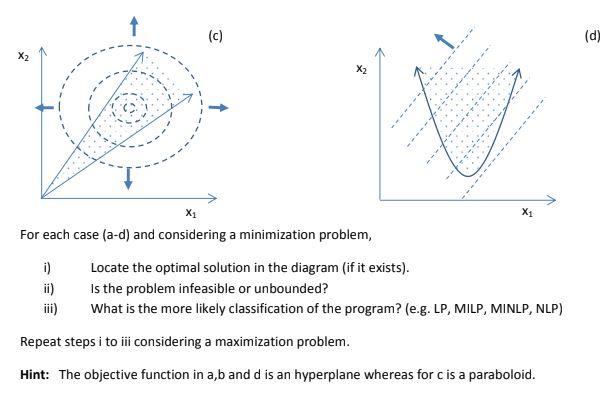# Question Solved1 AnswerAnswer only, if you are 100% sure otherwise will give you thumbs down. Problem 1 (CL: 10/NCL:15 )-The schematics (a) to (d) show the projection of mathematical programs onto the continuous space (note that the level curves of the objective function appear with dashed lines). The thick arrow represents the direction of increase of the objective function. (a) (b) X1 X1 1 ( (c) (d) X2 X2 X1 X1 For each case (a-d) and considering a minimization problem, i) Locate the optimal solution in the diagram (if it exists). ii) Is the problem infeasible or unbounded? iii) What is the more likely classification of the program? (e.g. LP, MILP, MINLP, NLP) Repeat steps i to iii considering a maximization problem. Hint: The objective function in a,b and d is an hyperplane whereas for c is a paraboloid.DTUVYQ The Asker · Chemical EngineeringAnswer only, if you are 100% sure otherwise will give you thumbs down.

Transcribed Image Text: Problem 1 (CL: 10/NCL:15 )-The schematics (a) to (d) show the projection of mathematical programs onto the continuous space (note that the level curves of the objective function appear with dashed lines). The thick arrow represents the direction of increase of the objective function. (a) (b) X1 X1 1 ( (c) (d) X2 X2 X1 X1 For each case (a-d) and considering a minimization problem, i) Locate the optimal solution in the diagram (if it exists). ii) Is the problem infeasible or unbounded? iii) What is the more likely classification of the program? (e.g. LP, MILP, MINLP, NLP) Repeat steps i to iii considering a maximization problem. Hint: The objective function in a,b and d is an hyperplane whereas for c is a paraboloid.
More
Transcribed Image Text: Problem 1 (CL: 10/NCL:15 )-The schematics (a) to (d) show the projection of mathematical programs onto the continuous space (note that the level curves of the objective function appear with dashed lines). The thick arrow represents the direction of increase of the objective function. (a) (b) X1 X1 1 ( (c) (d) X2 X2 X1 X1 For each case (a-d) and considering a minimization problem, i) Locate the optimal solution in the diagram (if it exists). ii) Is the problem infeasible or unbounded? iii) What is the more likely classification of the program? (e.g. LP, MILP, MINLP, NLP) Repeat steps i to iii considering a maximization problem. Hint: The objective function in a,b and d is an hyperplane whereas for c is a paraboloid.